Latest Banking jobs   »   Time & Distance

# Time & Distance Formula, Tricks, Question & Answer

The time & Distance formula is used in mathematics to determine the distance, time & Speed. This is one of the most important topics from which questions are asked in all the competitive exams like SSC, Railway, State PSC, CTET, Banking, and UPSC. The concept of time and distance is used by all of us in our day-to-day life as well therefore we are familiar with it. If we talk specifically about banking exams then the concept of time & distance is used by the examiner in the data interpretation questions to increase the difficulty level of the questions in the main examination. So today in this post, we are going to discuss all the important formulas required to solve Time & Distance questions along with the all other necessary basic concepts related to Time & Distance.

## Time & Distance

Let us first understand what distance and speed mean and then we will look at the basic formulas of time & distance.

Distance- It signifies how far objects are and measured in various units. It actually tells us how far an object is from us. It is numerical in nature along with its unit. The unit which is mostly used in time & distance questions is kilometer as well as meter.

Speed- Speed is a measure of change of position of something with respect to time. We can calculate speed after dividing distance with time. The unit of speed is kilometer per hour(Km/hr) as well as meter per second(m/s)

Time- Time can be defined in hour, minutes and seconds.

Here we have given below the basic formulas of the topic time and distance

• Distance= Speed x Time
• Time= Distance/Speed
• Speed= Distance/Time
• Average Speed=Total Distance Covered/Total Time Taken

## Important Points Of Time & Distance

• Time and speed are inversely proportional when the distance is constant. Two quantities are said to be inversely proportional when an increase in one quantity bring a decrease in other quantity. So when we  increase speed the time to cover the distance gets decreased and when the speed is decreased the time to cover the distance gets increased. If we have a ratio of the speed of two quantities is A:B then the ratio of speed will be B:A
• Speed is directly proportional to distance. Two quantities are said to be directly proportional when an increase in  quantity brings a increase in another quantity and a decrease in one quantity leads to decrease in another quantity

## Time & Distance Concepts: Tips & Tricks

In order to solve time & distance questions quickly, candidates must be aware of all the concepts which are used to solve the problems.

Conversion of Kilometer per hour into meter per second

X Km/hr= (X*5/18) m/s

Application of concept- Suppose you have given a speed of 90km/hr and now you want to convert it in m/s then multiply 90 by 5/18 and you will get your answer.

90 Km/hr= 90*5/18 m/s= 25 m/s

Conversion of meter per second into kilometer per hour

X M/s= (X*18/5) Km/hr

Application of concept- Suppose you have a given a speed of 20 m/s and now you want to convert it in Km/hr then multiply 20 by 18/5 and you will get your answer.

20 M/s= 20*18/5 Km/hr= 72 Km/hr

When two objects are moving in the opposite direction then relative speed will be

Relative speed= S1+S2

When two objects are moving in the same direction then relative speed will be

Relative speed= S2 – S1

If two persons C and D start at the same time from two points N and O towards each other and after crossing they take T1 and T2 hours in reaching O and N respectively, then

(C’s speed) / (D’s speed) = √T2 / √T1

When distance is equal

When d1 = d2, Average Speed = 2S1S2/(S1+S2), where S1 and S2 are the speeds for covering d1 and d2 respectively.

## Types of Questions in Time & Distance

### Races

A race involves runners covering a certain distance and the person who reaches the finishing point first wins the race. A ‘kilometer race’ requires the participants to run for 1 km. Unless otherwise specified, a race is assumed to be run on a linear track. A race can also be run on a circular track.

Suppose two runners A and B run a race.

• If A reaches the finishing point first and B is still behind by ‘x’ meters, A is said to win the race by ‘x’ metes. In this case, both ran for equal amounts of time but only A ran the whole distance.
• If A reaches the finishing point first and B needs ‘t’ more minutes to complete the race, A is said to win the race by ‘t’ minutes. In this case, both ran for equal distances but B took more time than A.
• If B starts running only ‘t’ minutes after A has started, A is said to have a start of ‘t’ minutes.
• If B starts running only after A has covered ‘x’ meters, A is said to have a start of ‘x’ meters.

### When Reaching Early & Late

In this kind of problem of time & distance there will be an object moving with certain speed and reaching late at its destination by some minutes but when moving with an increased speed which is more than  the previous speed then it gets late by the time which is less than the previous one. The formula which you can use to solve this problem quickly is given below. In case of late reduce the time difference will be taken

D=S1×S2/S2-S1×T2-T1

If a train runs at 40 kmph, it reaches its destination late by 11 minutes but if it runs at 50 kmph, it is late by 5 minutes only. The correct time for the train to complete its journey is

Sol. The distance will be 40×50/50-40×11-5/60= 20km

Speed=Distance/time

40=20/time

Time= 1/2×60= 30 minutes

The correct time for the train to complete its journey is 30 minutes – 11 minutes= 19 minutes

### Overtaking, Passing Bodies

Problems may often feature bodies that move while other bodies may move or remain stationary. In such cases, the following points are to be noted.

• When two bodies pass each other (one body may be stationary), the speed of passing is equal to the relative speed between the two bodies.
• Objects like man, car, cycle, telegraph pole and tree are to be taken as point objects, with negligible length. When a train of length ‘l’ passes such an object, the distance covered while passing is equal to the length of the train ‘l’.
• Objects like train, platform and bridge have length which needs to be taken into account when approaching the problem. When a train passes such an object of length ‘p’, the distance covered while passing is equal to the total length of the train and the object, i.e., ‘l + p’. This holds true even when the second object is another train.

### Travel & Meeting Problems

When two persons start from two points at the same time and travel towards each other, the time taken by each of them to reach the meeting point is the same. Hence, the distances covered by them from their respective starting points to the meeting point will be proportional to their respective speeds.### Boats and Streams based Problems

The two quantities that come into the picture are the speed of the stream or river or current, and the speed of the boat or swimmer in still water.

• When the boat is moving against the current, it is said to move ‘upstream’. Then the effective speed at which it moves is the difference between its speed in still water and the speed of the current.
• When the boat is moving with the current, it is said to move ‘downstream’. Then the effective speed at which it moves is the sum of its speed in still water and the speed of the current.

If u and v are the upstream and downstream speeds of the boat, then

• Speed of the boat in still water = (u + v)/2
• Speed of the stream = (v – u)/2

## Time & Distance

Q1. A train crosses a man, who is running in the same direction of train at the speed of 2m/sec. in 10 seconds. The same train crosses a tunnel in 54 seconds. If speed of train is 72 km/h then what is the length of tunnel?
(a) 850 m
(b) 800 m
(c) 900 m
(d) 750 m
(e) 650 m

Q2. Raghav can beat Suresh by 100m in a race of 1 km and they run at speed of 10m/sec and 8 m/sec respectively. If Suresh increases his speed by 7m/sec then by how much time he will beat Raghav in the same race of 1km?
(a) 43 sec
(b) 36 sec
(c) 110/3 sec
(d) 100/3 sec
(e) 70/3 sec

Q3. A boat can cover upstream distance between two points in 6 hours and same distance downstream in 4 hours. If speed of boat in still water is 8 km/h, then find speed of stream.
(a) 2 km/h
(b) 1.6 km/h
(c) 3.2 km/h
(d) 4.8 km/h
(e) 3.8 km/h

Q4. Two trains X and Y cross each other in 48 sec, when both are running in same direction. If length of train X is 160 m and speed of train X and train Y are 54 km/h and 72 km/h respectively then find the length of train Y.
(a) 160 m
(b) 120 m
(c) 80 m
(d) 100 m
(e) 95 cm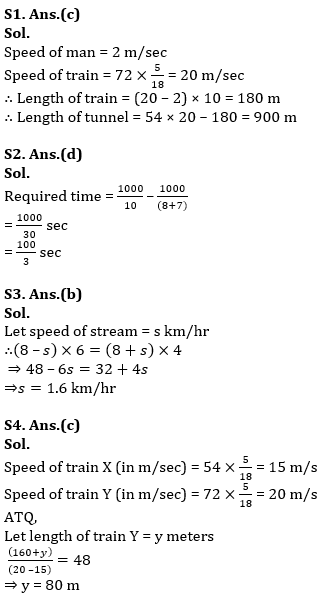Q5. A train P running with speed 54 kmph crosses a man in 30 sec. In how much time it can cross a 180 m long platform.
(a) 51 sec
(b) 45 sec
(c) 42 sec
(d) 39 sec
(e) 58 sec

Q6. A boat can travel with the speed of 17 kmph in upstream. If the speed of river is 3 kmph, then find the speed of boat in downstream in the same river.
(a) 23 kmph
(b) 20 kmph
(c) 25 kmph
(d) 19 kmph
(e) 21 kmph

Q7. A 570 m long train can cross a pole in 38 sec. In how much time it can cross a 660 m long platform.
(a) 82 sec
(b) 64 sec
(c) 90 sec
(d) 120 sec
(e) 72 sec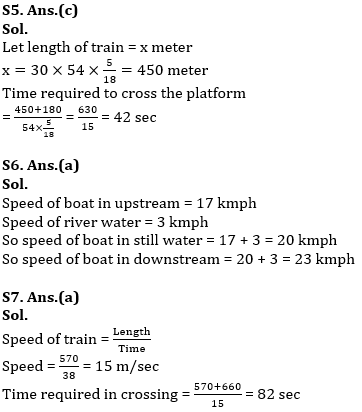Q8. Ratio between length of trains A and B is 3 : 5. Speed of train A is 72 km/h and that of train B is 54 km/h & they are running opposite to each other. If train A cross’s train B in 16 seconds then find length of train B.
(a) 350 m
(b) 250 m
(c) 450 m
(d) 150 m
(e) 320 m

Q9. Speed of a boat in still water is 8 km/h. It takes 5 hours to go upstream and 3 hours downstream distance between two points. What is the speed of stream?
(a) 4 km/h
(b) 2 km/h
(c) 3 km/h
(d) 1 km/h
(e) 2.5 km/h

Q10. Speed of a train is 90 km/h. It crosses a platform and a pole in 36 seconds and 6 seconds respectively. Find the length of platform.
(a) 450m
(b) 650 m
(c) 750m
(d) 850m
(e) 550m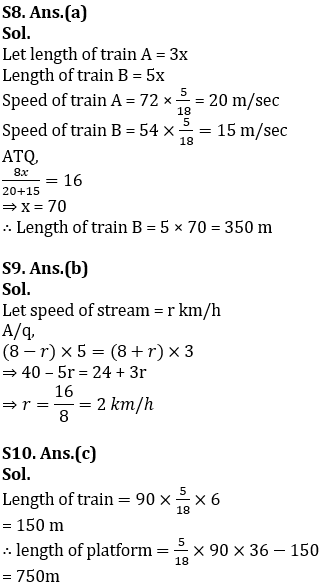Q11. A man covers half of total distance with 12 km/h and another half distance with 24km/h. Find his average speed.
(a) 12 km/h
(b) 16 km/h
(c) 10 km/h
(d) 18 km/h
(e) 6 km/h

Q12. The ratio of speed of two trains which are running in the same direction is 4 : 5. The train having higher speed crosses the second train in 30 seconds and a pole in 4 seconds respectively. Find the ratio of their lengths.
(a) 1 : 2
(b) 2 : 3
(c) 3 : 5
(d) 4 : 5
(e) 6 : 7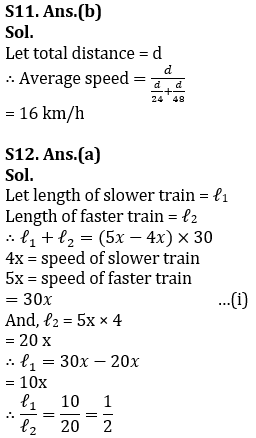Q13. Speed of a boat in still water is 300% more than the speed of current. The boat takes a total time of 8 hours to cover a distance of 45 km upstream and 45 km in downstream both. Find speed of current.
(a) 2.5 km/h
(b) 2 km/h
(c) 4 km/h
(d) 3 km/h
(e) 5 km/h

Q14. Two busses start from Delhi to Karnal at 8 am and 10 am respectively. Speed of bus starting at 8 a.m. and speed of bus starting at 10 a.m. is 20 m/sec and 25 m/sec respectively. If distance between Delhi to Karnal is 200 km then at what time faster bus will catch the slower bus?
(a) 8h
(b)10 h
(c) 12 h
(d) 6 h
(e) 14 h

Q15. Ratio of the speeds of Ajay and Ramesh is 2 : 5. If Ajay covers 240 km in 8hrs then in how much time Ramesh will cover 780 km of distance?
(a) 10hrs 24minutes
(b) 5 hrs 48 minutes
(c) 8 hrs 40minutes
(d) 12 hrs 20 minutes
(e) 10 hrs and 36 minutes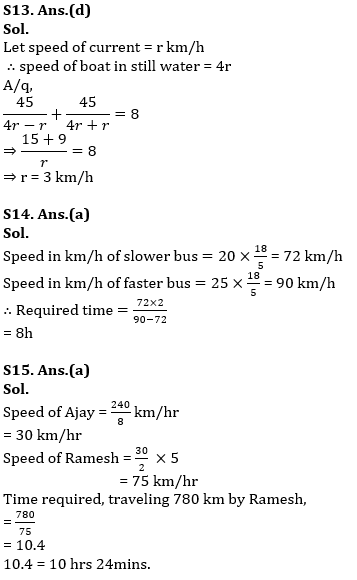## FAQs: Time & Distance

Q.1 What is the formula to calculate distance?
Ans The formula to calculate distance is speed multiplied by time

Q.2 What are the basic concepts required to solve the time & distance questions?
Ans The basic concepts required to solve the time & distance questions is given in the article above

Q.3 Where will I get time & distance questions for practice?
Ans You can practice time & distance questions from adda247 app in which questions are available in the form of quizzes

Current Affairs## FAQs

### Q.1 What is the formula to calculate distance?

Ans The formula to calculate distance is speed multiplied by time

### Q.2 What are the basic concepts required to solve the time & distance questions?

Ans The basic concepts required to solve the time & distance questions is given in the article above

### Q.3 Where will I get time & distance questions for practice?

Ans You can practice time & distance questions from adda247 app which are available in the form of quizzes

#### Congratulations!Union Budget 2023-24: Free PDF